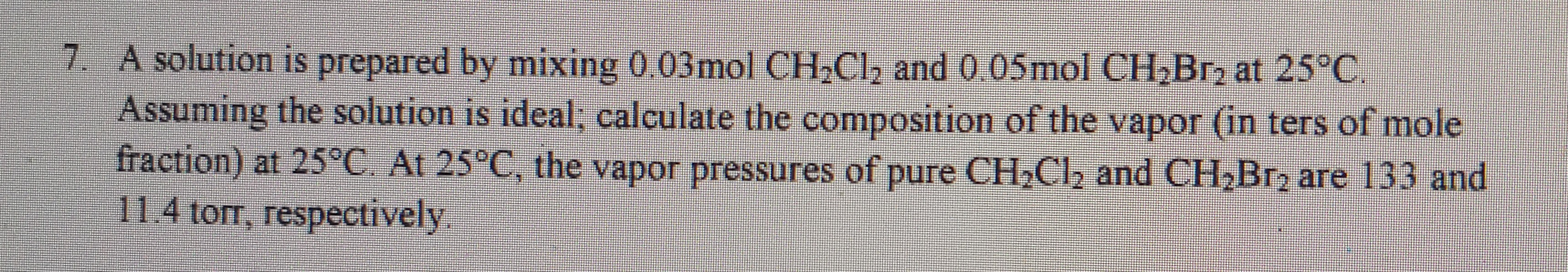# A solution is prepared by mixing 0.03 mol CH2Cl2 and .05 mol Ch2Br2 at 25C. Assuming the solution is ideal; calculate the composition of the vapor (in terms of mole fraction) at 25C. At 25C, the vapor pressures of pure CH2Cl2 and CH2Br2 are 133 and 11.4 torr, respectively